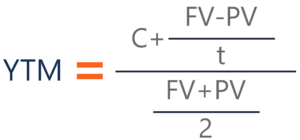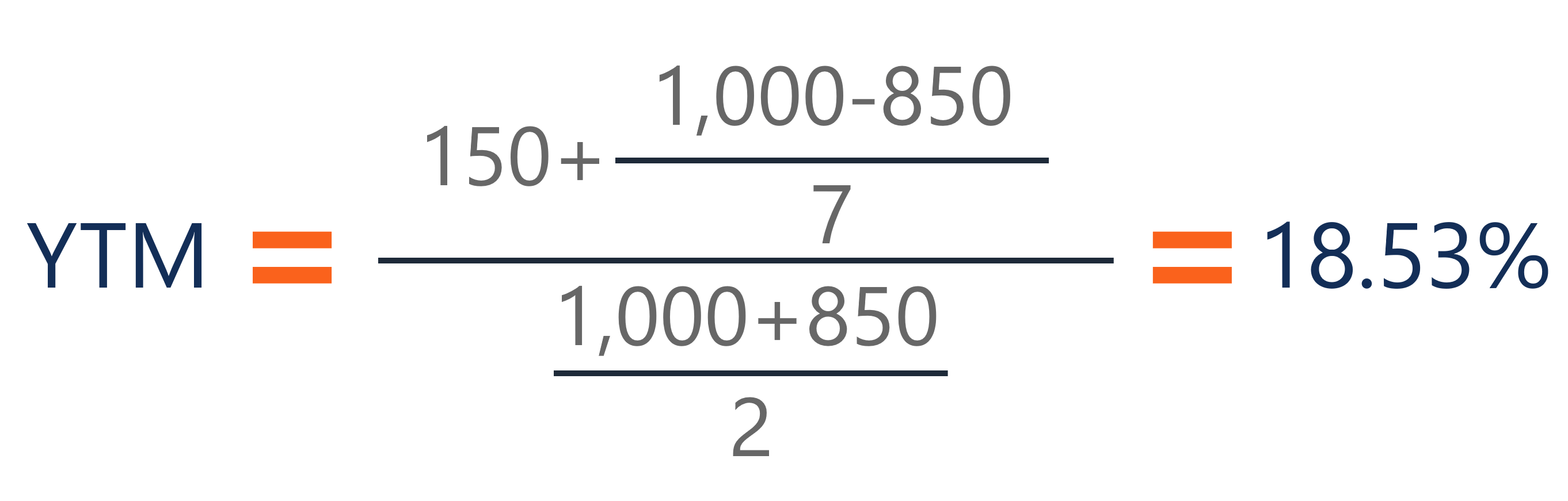# Yield to Maturity (YTM)

The speculative rate of return or interest rate of a fixed-rate security

## What is the Yield to Maturity (YTM)?

Yield to Maturity (YTM) – otherwise referred to as redemption or book yield – is the speculative rate of return or interest rate of a fixed-rate security, such as a bond. The YTM is based on the belief or understanding that an investor purchases the security at the current market price and holds it until the security has matured (reached its full value), and that all interest and coupon payments are made in a timely fashion.### How YTM is Calculated

YTM is typically expressed as an annual percentage rate (APR). It is determined through the use of the following formula:Where:

• C – Interest/coupon payment
• FV – Face value of the security
• PV – Present value/price of the security
• t – How many years it takes the security to reach maturity

The formula’s purpose is to determine the yield of a bond (or other fixed-asset security) according to its most recent market price. The YTM calculation is structured to show – based on compounding – the effective yield a security should have once it reaches maturity. It is different from simple yield, which determines the yield a security should have upon maturity, but is based on dividends and not compounded interest.

### Approximated YTM

It’s important to understand that the formula above is only useful for an approximated YTM. In order to calculate the true YTM, an analyst or investor must use the trial and error method. It is done by using a variety of rates that are substituted into the current value slot of the formula. The true YTM is determined once the price matches that of the security’s actual current market price.

Alternatively, this process can be sped up by utilizing the SOLVER function in Excel, which determines a value based on conditions that can be set. This means that an analyst can set the present value (price) of the security and solve for the YTM which acts as the interest rate for the PV calculation.

### Example of a YTM Calculation

To get a better understanding of the YTM formula and how it works, let’s look at an example.

Assume that there is a bond on the market priced at \$850 and that the bond comes with a face value of \$1,000 (a fairly common face value for bonds). On this bond, yearly coupons are \$150. The coupon rate for the bond is 15% and the bond will reach maturity in 7 years.

The formula for determining approximate YTM would look like below:The approximated YTM on the bond is 18.53%.

### Importance of Yield to Maturity

The primary importance of yield to maturity is the fact that it enables investors to draw comparisons between different securities and the returns they can expect from each. It is critical for determining which securities to add to their portfolios. It’s also useful in that it also allows the investors to gain some understanding of how changes in market conditions might affect their portfolio because when securities drop in price, yields rise, and vice versa.

CFI is the official provider of the Financial Modeling and Valuation Analyst (FMVA)™ certification program, designed to transform anyone into a world-class financial analyst. If you are looking to learn more about fixed income securities, check out some of the resources below!

• Fixed Income Fundamentals
• Equity vs Fixed Income
• Held to Maturity Securities
• Matrix Pricing

### Financial Analyst Training

Get world-class financial training with CFI’s online certified financial analyst training program!

Gain the confidence you need to move up the ladder in a high powered corporate finance career path.

Learn financial modeling and valuation in Excel the easy way, with step-by-step training.